The GLIMMIX Procedure
 ESTIMATE Statement
ESTIMATE ’label’ contrast-specification <(divisor=n)>
<, ’label’ contrast-specification <(divisor=n)> > <, ...>
< / options>
;
The ESTIMATE statement provides a mechanism for obtaining custom hypothesis tests. As in the CONTRAST statement, the basic element of the ESTIMATE statement is the contrast-specification, which consists of MODEL and G-side random effects and their coefficients. Specifically, a contrast-specification takes the form
< fixed-effect values ...> < | random-effect values ...>

Based on the contrast-specifications in your ESTIMATE statement, PROC GLIMMIX constructs the matrix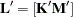, as in the CONTRAST statement, where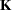is associated with the fixed effects and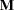is associated with the G-side random effects. The GLIMMIX procedure supports nonpositional syntax for the coefficients of fixed effects in the ESTIMATE statement. For details see the section Positional and Nonpositional Syntax for Contrast Coefficients.

PROC GLIMMIX then produces for each rowof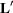an approximate t test of the hypothesis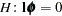, where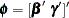. You can also obtain multiplicity-adjusted p-values and confidence limits for multirow estimates with the ADJUST= option. The output from multiple ESTIMATE statements is organized as follows. Results from unadjusted estimates are reported first in a single table, followed by separate tables for each of the adjusted estimates. Results from all ESTIMATE statements are combined in the "Estimates" ODS table.

Note that multirow estimates are permitted. Unlike the CONTRAST statement, you need to specify a ’label’ for every row of the multirow estimate, because PROC GLIMMIX produces one test per row.

PROC GLIMMIX selects the degrees of freedom to match those displayed in the "Type III Tests of Fixed Effects" table for the final effect you list in the ESTIMATE statement. You can modify the degrees of freedom by using the DF= option. If you select DDFM=NONE and do not modify the degrees of freedom by using the DF= option, PROC GLIMMIX uses infinite degrees of freedom, essentially computing approximate z tests. If PROC GLIMMIX finds the fixed-effects portion of the specified estimate to be nonestimable, then it displays "Non-est" for the estimate entry.

specifies how denominator degrees of freedom are determined when p-values and confidence limits are adjusted for multiple comparisons with the ADJUST= option. When you do not specify the ADJDFE= option, or when you specify ADJDFE=SOURCE, the denominator degrees of freedom for multiplicity-adjusted results are the denominator degrees of freedom for the final effect listed in the ESTIMATE statement from the "Type III Tests of Fixed Effects" table.

The ADJDFE=ROW setting is useful if you want multiplicity adjustments to take into account that denominator degrees of freedom are not constant across estimates. This can be the case, for example, when the DDFM=SATTERTHWAITE or DDFM=KENWARDROGER degrees-of-freedom method is in effect.

requests a multiple comparison adjustment for the p-values and confidence limits for the estimates. The adjusted quantities are produced in addition to the unadjusted quantities. Adjusted confidence limits are produced if the CL or ALPHA= option is in effect. For a description of the adjustments, see Chapter 39, The GLM Procedure, and Chapter 58, The MULTTEST Procedure, of the SAS/STAT User’s Guide and the documentation for the ADJUST= option in the LSMEANS statement. The ADJUST= option is ignored for generalized logit models.

If the STEPDOWN option is in effect, the p-values are further adjusted in a step-down fashion.

ALPHA=number

requests that a t-type confidence interval be constructed with confidence level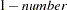. The value of number must be between 0 and 1; the default is 0.05. If DDFM=NONE and you do not specify degrees of freedom with the DF= option, PROC GLIMMIX uses infinite degrees of freedom, essentially computing a z interval.

BYCATEGORY
BYCAT

requests that in models for nominal data (generalized logit models) estimates be reported separately for each category. In contrast to the BYCATEGORY option in the CONTRAST statement, an ESTIMATE statement in a generalized logit model does not distribute coefficients by response category, because ESTIMATE statements always correspond to single rows of the
matrix.

For example, assume that the response variable Style is multinomial with three (unordered) categories. The following GLIMMIX statements fit a generalized logit model relating the preferred style of instruction to school and educational program effects:

```   proc glimmix data=school;
class School Program;
model Style(order=data) = School Program / s ddfm=none
freq Count;
estimate 'School 1 vs. 2' school 1 -1 / bycat;
estimate 'School 1 vs. 2' school 1 -1;
run;
```

The first ESTIMATE statement compares school effects separately for each nonredundant category. The second ESTIMATE statement compares the school effects for the first non-reference category.

The BYCATEGORY option has no effect unless your model is a generalized (mixed) logit model.

CL

requests that t-type confidence limits be constructed. If DDFM=NONE and you do not specify degrees of freedom with the DF= option, PROC GLIMMIX uses infinite degrees of freedom, essentially computing a z interval. The confidence level is 0.95 by default. These intervals are adjusted for multiplicity when you specify the ADJUST= option.

DF=number

specifies the degrees of freedom for the t test and confidence limits. The default is the denominator degrees of freedom taken from the "Type III Tests of Fixed Effects" table and corresponds to the final effect you list in the ESTIMATE statement.

DIVISOR=value-list

specifies a list of values by which to divide the coefficients so that fractional coefficients can be entered as integer numerators. If you do not specify value-list, a default value of 1.0 is assumed. Missing values in the value-list are converted to 1.0.

If the number of elements in value-list exceeds the number of rows of the estimate, the extra values are ignored. If the number of elements in value-list is less than the number of rows of the estimate, the last value in value-list is copied forward.

If you specify a row-specific divisor as part of the specification of the estimate row, this value multiplies the corresponding divisor implied by the value-list. For example, the following statement divides the coefficients in the first row by 8, and the coefficients in the third and fourth row by 3:

```   estimate 'One vs. two'   A 2 -2  (divisor=2),
'One vs. three' A 1  0 -1         ,
'One vs. four'  A 3  0  0 -3      ,
'One vs. five'  A 1  0  0  0  -1  / divisor=4,.,3;
```

Coefficients in the second row are not altered.

E

requests that thematrix coefficients be displayed.

EXP

requests exponentiation of the estimate. When you model data with the logit, cumulative logit, or generalized logit link functions, and the estimate represents a log odds ratio or log cumulative odds ratio, the EXP option produces an odds ratio. See Odds and Odds Ratio Estimation for important details about the computation and interpretation of odds and odds ratio results with the GLIMMIX procedure. If you specify the CL or ALPHA= option, the (adjusted) confidence bounds are also exponentiated.

GROUP coeffs

sets up random-effect contrasts between different groups when a GROUP= variable appears in the RANDOM statement. By default, ESTIMATE statement coefficients on random effects are distributed equally across groups. If you enter a multirow estimate, you can also enter multiple rows for the GROUP coefficients. If the number of GROUP coefficients is less than the number of contrasts in the ESTIMATE statement, the GLIMMIX procedure cycles through the GROUP coefficients. For example, the following two statements are equivalent:

```   estimate 'Trt 1 vs 2 @ x=0.4' trt 1 -1  0 | x 0.4,
'Trt 1 vs 3 @ x=0.4' trt 1  0 -1 | x 0.4,
'Trt 1 vs 2 @ x=0.5' trt 1 -1  0 | x 0.5,
'Trt 1 vs 3 @ x=0.5' trt 1  0 -1 | x 0.5 /
group 1 -1, 1 0 -1, 1 -1, 1 0 -1;

estimate 'Trt 1 vs 2 @ x=0.4' trt 1 -1  0 | x 0.4,
'Trt 1 vs 3 @ x=0.4' trt 1  0 -1 | x 0.4,
'Trt 1 vs 2 @ x=0.5' trt 1 -1  0 | x 0.5,
'Trt 1 vs 3 @ x=0.5' trt 1  0 -1 | x 0.5 /
group 1 -1, 1 0 -1;
```

requests that the estimate and its standard error are also reported on the scale of the mean (the inverse linked scale). PROC GLIMMIX computes the value on the mean scale by applying the inverse link to the estimate. The interpretation of this quantity depends on the fixed-effect values and random-effect values specified in your ESTIMATE statement and on the link function. In a model for binary data with logit link, for example, the following statements compute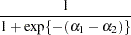where
and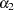are the fixed-effects solutions associated with the first two levels of the classification effect A:

```   proc glimmix;
class A;
model y = A / dist=binary link=logit;
estimate 'A one vs. two' A 1 -1 / ilink;
run;
```

This quantity is not the difference of the probabilities associated with the two levels,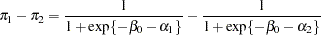The standard error of the inversely linked estimate is based on the delta method. If you also specify the CL option, the GLIMMIX procedure computes confidence limits for the estimate on the mean scale. In multinomial models for nominal data, the limits are obtained by the delta method. In other models they are obtained from the inverse link transformation of the confidence limits for the estimate. The ILINK option is specific to an ESTIMATE statement.

LOWER
LOWERTAILED

requests that the p-value for the t test be based only on values less than the test statistic. A two-tailed test is the default. A lower-tailed confidence limit is also produced if you specify the CL or ALPHA= option.

Note that for ADJUST=SCHEFFE the one-sided adjusted confidence intervals and one-sided adjusted p-values are the same as the corresponding two-sided statistics, because this adjustment is based on only the right tail of the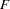distribution.

SINGULAR=number

tunes the estimability checking as documented for the CONTRAST statement..

STEPDOWN<(step-down-options)>

requests that multiplicity adjustments for the p-values of estimates be further adjusted in a step-down fashion. Step-down methods increase the power of multiple testing procedures by taking advantage of the fact that a p-value will never be declared significant unless all smaller p-values are also declared significant. Note that the STEPDOWN adjustment combined with ADJUST=BON corresponds to the methods of Holm (1979) and Shaffer’s "Method 2" (1986); this is the default. Using step-down-adjusted p-values combined with ADJUST=SIMULATE corresponds to the method of Westfall (1997).

If the degrees-of-freedom method is DDFM=KENWARDROGER or DDFM=SATTERTHWAITE, then step-down-adjusted p-values are produced only if the ADJDFE=ROW option is in effect.

Also, the STEPDOWN option affects only p-values, not confidence limits. For ADJUST=SIMULATE, the generalized least squares hybrid approach of Westfall (1997) is employed to increase Monte Carlo accuracy.

You can specify the following step-down-options in parentheses after the STEPDOWN option.

MAXTIME=n

specifies the time (in seconds) to spend computing the maximal logically consistent sequential subsets of equality hypotheses for TYPE=LOGICAL. The default is MAXTIME=60. If the MAXTIME value is exceeded, the adjusted tests are not computed. When this occurs, you can try increasing the MAXTIME value. However, note that there are common multiple comparisons problems for which this computation requires a huge amount of time—for example, all pairwise comparisons between more than 10 groups. In such cases, try to use TYPE=FREE (the default) or TYPE=LOGICAL() for small.

ORDER=PVALUE
ORDER=ROWS

specifies the order in which the step-down tests are performed. ORDER=PVALUE is the default, with estimates being declared significant only if all estimates with smaller (unadjusted) p-values are significant. If you specify ORDER=ROWS, then significances are evaluated in the order in which they are specified in the syntax.

REPORT

specifies that a report on the step-down adjustment be displayed, including a listing of the sequential subsets (Westfall 1997) and, for ADJUST=SIMULATE, the step-down simulation results.

TYPE=LOGICAL<(n)>
TYPE=FREE

If you specify TYPE=LOGICAL, the step-down adjustments are computed by using maximal logically consistent sequential subsets of equality hypotheses (Shaffer 1986, Westfall 1997). Alternatively, for TYPE=FREE, sequential subsets are computed ignoring logical constraints. The TYPE=FREE results are more conservative than those for TYPE=LOGICAL, but they can be much more efficient to produce for many estimates. For example, it is not feasible to take logical constraints between all pairwise comparisons of more than about 10 groups. For this reason, TYPE=FREE is the default.

However, you can reduce the computational complexity of taking logical constraints into account by limiting the depth of the search tree used to compute them, specifying the optional depth parameter as a numberin parentheses after TYPE=LOGICAL. As with TYPE=FREE, results for TYPE=LOGICAL() are conservative relative to the true TYPE=LOGICAL results, but even for TYPE=LOGICAL() they can be appreciably less conservative than TYPE=FREE and they are computationally feasible for much larger numbers of estimates. If you do not specifyor if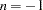, the full search tree is used.

SUBJECT coeffs

sets up random-effect contrasts between different subjects when a SUBJECT= variable appears in the RANDOM statement. By default, ESTIMATE statement coefficients on random effects are distributed equally across subjects. Listing subject coefficients for an ESTIMATE statement with multiple rows follows the same rules as for GROUP coefficients.

UPPER
UPPERTAILED

requests that the p-value for the t test be based only on values greater than the test statistic. A two-tailed test is the default. An upper-tailed confidence limit is also produced if you specify the CL or ALPHA= option.

Note that for ADJUST=SCHEFFE the one-sided adjusted confidence intervals and one-sided adjusted p-values are the same as the corresponding two-sided statistics, because this adjustment is based on only the right tail of thedistribution.Previous Page | Next Page | Top of Page